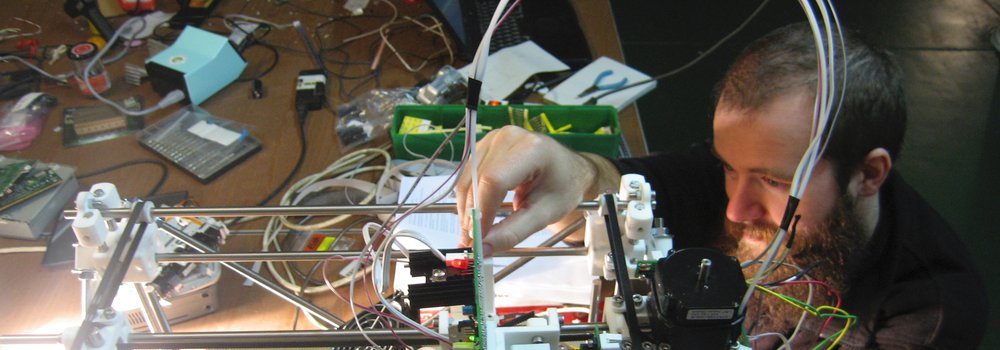# Residency Steven Pickles (Pix)this activity is archived
freshness:

In 2001 Pix found a book by mathematician Julien C. Sprott called Strange Attractors: Creating Patterns in Chaos.  The book described the mathematics behind a class of fractals called strange attractors. In the book, Sprott presents a computer program which can search for strange attractors by trying many randomly generated equations until one is discovered which meets certain criteria. The results are clouds of points which form interesting organic shapes. each set of equations created a unique set of points. When Pix read this, he was curious to see what would be the outcome of making small changes to the equations. He made a simple program which did this, and the result was clouds of points which would morph between many different shapes. The program would move through the parameter space randomly and automatically, and so it only showed that there was some kind of continuity to the parameter space which the strange attractors occupied, but it did not provide any useful way of visualising this space. In this research Pix explored this space, and maped it to some degree.